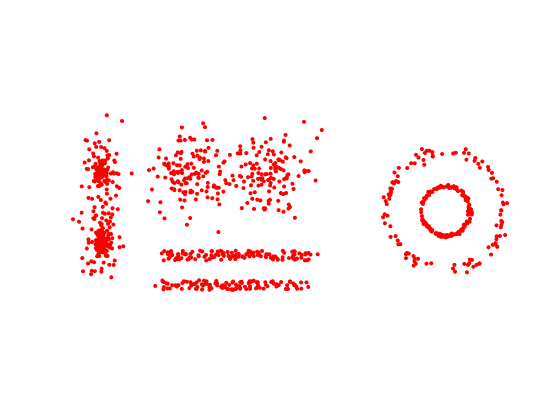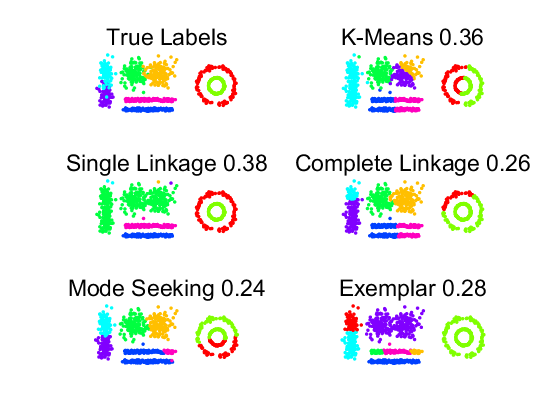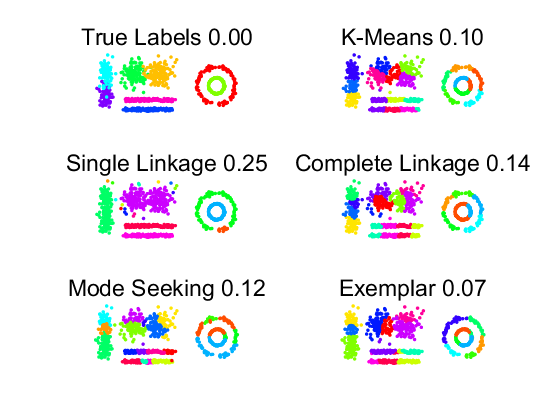# ct_intro: Introduction of clustering by ClusterTools

PRTools and ClusterTools should be in the path

## Prepare environment

```prtime(10)                   % restrict iterative optimisation to 10s
delfigs                      % delete existing figures
randreset;                   % takes care of reproducability
prwarning(2)                 % show warnings
```

## Define a dataset

we use some standard routines to create 8 two-dimensional clusters. After creation the label information is removed.

```m = 1000;
a = gendatclust2(m);
x = +a; % remove labels
scattern(x); axis equal
```This dataset consist of:

• two straight, noisy lines
• two noisy circles inside each other
• two spherical normal distributions
• two elongated non-normal distributions.

## Find 8 clusters by five routines

• Find 8 clusters.
• Compare with ground truth.
• Display active learning error, i.e. the error if the entire clusters are assigned to the prototype class.

First a separate coding per cluster routine is shown. In the bottom experiment a more compact coding is used.

```figure;
labt = getnlab(a);
subplot(3,2,1); scatn(labt,x,'True Labels');
markcols(1);
axis equal; drawnow

labk = clustk(x,8); e = clusteval(labk,a,'actl');
title = ['K-Means ' num2str(e,'%5.2f')];
subplot(3,2,2); scatn(labk,x,title);
markcols(1);
axis equal; drawnow

labhs = clusth(x,8,'s'); e = clusteval(labhs,a,'actl');
title = ['Single Linkage ' num2str(e,'%5.2f')];
subplot(3,2,3); scatn(labhs,x,title);
markcols(1);
axis equal; drawnow

labhc = clusth(x,8,'c'); e = clusteval(labhc,a,'actl');
title = ['Complete Linkage ' num2str(e,'%5.2f')];
subplot(3,2,4); scatn(labhc,x,title);
markcols(1);
axis equal; drawnow

labm = clustm(x,8); e = clusteval(labm,a,'actl');
title = ['Mode Seeking ' num2str(e,'%5.2f')];
subplot(3,2,5); scatn(labm,x,title);
markcols(1);
axis equal; drawnow

labe = cluste(x,8); e = clusteval(labe,a,'actl');
title = ['Exemplar ' num2str(e,'%5.2f')];
subplot(3,2,6); scatn(labe,x,title);
markcols(1);
axis equal; drawnow
clear title
```
```PR_Warning: EXEMPLAR: Examplar clustering updating stopped by PRTIME after 202 iterations
```K-Means, Complete Linkage and Mode Seeking result in similar clusters, that are about spherical and of the same size. Single Linkage shows an interesting result as it finds the two bars as well as the two circles as single clusters, but it merges the four Gaussian blobs. In addition there are three single object clusters. Exemplar performs badly. Note that it generated a warning as its optimisation needed too much time.

## Find 20 clusters by five routines

• Find 20 clusters.
• Compare with ground truth.
• Display active learning error, i.e. the error if the entire clusters are assigned to the prototype class.

Coding here is more comapact than above. It uses the fact that the cluster routines are programmed as PRTools mappings.

```figure;
k = 20;
w = {clustk(k) clusth(k,'s') clusth(k,'c') clustm(k) cluste(k)};
'Mode Seeking','Exemplar'};
for i=1:6
% for i==1 a trick is used to get ClusterTools labels from dataset
if i == 1, lab = getnlab(a)*clust2proto(a); e = 0;
else lab = x*w{i-1}; e = clusteval(lab,a,'actl'); end
title = [names{i} ' ' num2str(e,'%5.2f')];
subplot(3,2,i); scatn(lab,x,title); markcols(1);
axis equal; drawnow
end
```
```PR_Warning: EXEMPLAR: Examplar clustering updating stopped by PRTIME after 204 iterations
```As there are more clusters than in the first experiment (20 instead of 8) for more objects the true labels are used. These are assigned to all objects in the corresponding cluster, resulting in a better perforemance. Exemplar now performs well. Frequently it is good (but very time consuming) for larger datasets and more clusters.